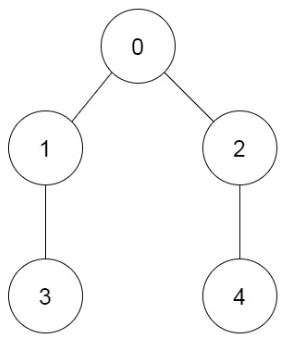# Count Ways to Build Rooms in an Ant Colony Solution

Page Contents

## Count Ways to Build Rooms in an Ant Colony

You are an ant tasked with adding `n` new rooms numbered `0` to `n-1` to your colony. You are given the expansion plan as a 0-indexed integer array of length `n``prevRoom`, where `prevRoom[i]` indicates that you must build room `prevRoom[i]` before building room `i`, and these two rooms must be connected directly. Room `0` is already built, so `prevRoom = -1`. The expansion plan is given such that once all the rooms are built, every room will be reachable from room `0`.

You can only build one room at a time, and you can travel freely between rooms you have already built only if they are connected. You can choose to build any room as long as its previous room is already built.

Return the number of different orders you can build all the rooms in. Since the answer may be large, return it modulo `109 + 7`.

Example 1:

```Input: prevRoom = [-1,0,1]
Output: 1
Explanation: There is only one way to build the additional rooms: 0 → 1 → 2
```

Example 2:```Input: prevRoom = [-1,0,0,1,2]
Output: 6
Explanation:
The 6 ways are:
0 → 1 → 3 → 2 → 4
0 → 2 → 4 → 1 → 3
0 → 1 → 2 → 3 → 4
0 → 1 → 2 → 4 → 3
0 → 2 → 1 → 3 → 4
0 → 2 → 1 → 4 → 3
```

Constraints:

• `n == prevRoom.length`
• `2 <= n <= 105`
• `prevRoom == -1`
• `0 <= prevRoom[i] < n` for all `1 <= i < n`
• Every room is reachable from room `0` once all the rooms are built.

Explanation:

For each subtree, we keep track of

• The number of nodes `a`
• The number of ways to order `x`

• The number of nodes is `a+b+1`
• The 1 is the parent node.
• The number of ways is the product of
• The number of ways to order the first subtree `x`
• The number of ways to order the second subtree `y`
• The number of ways to insert the first order into the second order
• This is `a+b choose a`
• Functions were written to compute `a+b choose a mod p` efficiently

To add more than two children, you iteratively combine two subtrees and consider them to behave like one subtree.

Program:

``````M9 = 10**9 + 7
n = 10**5 + 10

# preprocess factorials
factorial_mod_p = 
val = 1
for i in range(1,n+1):
val = (val*i)%M9
factorial_mod_p.append(val)

def ncr_mod_p(n, r, p=M9):
num = factorial_mod_p[n]
dem = factorial_mod_p[r]*factorial_mod_p[n-r]
return (num * pow(dem, p-2, p))%p

def product(a,b,x,y):
res = (x*y)%M9
res = res%M9
res = res*ncr_mod_p(a+b, a)
res = res%M9
return res

class Solution:
def waysToBuildRooms(self, prevRoom: List[int]) -> int:

g = defaultdict(list)
for i,x in enumerate(prevRoom):
g[x].append(i)

def dfs(node):
children = g[node]
if children == []:
return 1,1

a,x = dfs(children)

for child in children[1:]:
b, y = dfs(child)
x = product(a,b,x,y)
a = a+b

return a+1, x

return dfs(-1)[-1]``````

Credit : Here